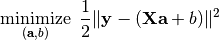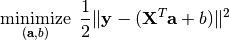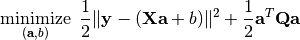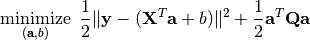Linear Least Square and Ridge Regression¶

The package provides functions to perform Linear Least Square and Ridge Regression.

Linear Least Square¶

Linear Least Square is to find linear combination(s) of given variables to fit the responses by minimizing the squared error between them. This can be formulated as an optimization as follows:Sometimes, the coefficient matrix is given in a transposed form, in which case, the optimization is modified as:The package provides llsq to solve these problems:

llsq(X, y; ...)

Solve the linear least square problem formulated above.

Here, y can be either a vector, or a matrix where each column is a response vector.

This function accepts two keyword arguments:

• trans: whether to use the transposed form. (default is false)
• bias: whether to include the bias term b. (default is true)

The function results the solution a. In particular, when y is a vector (matrix), a is also a vector (matrix). If bias is true, then the returned array is augmented as [a; b].

Examples

For a single response vector y (without using bias):

using MultivariateStats

# prepare data
X = rand(1000, 3)               # feature matrix
a0 = rand(3)                    # ground truths
y = X * a0 + 0.1 * randn(1000)  # generate response

# solve using llsq
a = llsq(X, y; bias=false)

# do prediction
yp = X * a

# measure the error
rmse = sqrt(mean(abs2(y - yp)))
print("rmse = \$rmse")

For a single response vector y (using bias):

# prepare data
X = rand(1000, 3)
a0, b0 = rand(3), rand()
y = X * a0 + b0 + 0.1 * randn(1000)

# solve using llsq
sol = llsq(X, y)

# extract results
a, b = sol[1:end-1], sol[end]

# do prediction
yp = X * a + b'

For a matrix Y comprised of multiple columns:

# prepare data
X = rand(1000, 3)
A0, b0 = rand(3, 5), rand(1, 5)
Y = (X * A0 .+ b0) + 0.1 * randn(1000, 5)

# solve using llsq
sol = llsq(X, Y)

# extract results
A, b = sol[1:end-1,:], sol[end,:]

# do prediction
Yp = X * A .+ b'

Ridge Regression¶

Compared to linear least square, Ridge Regression uses an additional quadratic term to regularize the problem:The transposed form:The package provides ridge to solve these problems:

ridge(X, y, r; ...)

Solve the ridge regression problem formulated above.

Here, y can be either a vector, or a matrix where each column is a response vector.

The argument r gives the quadratic regularization matrix Q, which can be in either of the following forms:

• r is a real scalar, then Q is considered to be r * eye(n), where n is the dimension of a.
• r is a real vector, then Q is considered to be diagm(r).
• r is a real symmetric matrix, then Q is simply considered to be r.

This function accepts two keyword arguments:

• trans: whether to use the transposed form. (default is false)
• bias: whether to include the bias term b. (default is true)

The function results the solution a. In particular, when y is a vector (matrix), a is also a vector (matrix). If bias is true, then the returned array is augmented as [a; b].